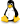.

# Zonal Statistics Plugin¶

With theZonal statistics plugin, you can analyze the results of a thematic classification. It allows you to calculate several values of the pixels of a raster layer with the help of a polygonal vector layer (see figure_zonal_statistics). You can calculate the sum, the mean value and the total count of the pixels that are within a polygon. The plugin generates output columns in the vector layer with a user-defined prefix.

Figure Zonal StatisticsZonal statistics dialog (KDE)